# Interest Rate Parity (IRP)

The relationship between the exchange rate and interest rates of two countries

## What is the Interest Rate Parity (IRP)?

The interest rate parity (IRP) is a theory that demonstrates the relationship between the spot exchange rate and the expected spot exchange rate or forward exchange rate of two countries, based on interest rates. This relationship says that the forward exchange rate should be equal to the spot exchange rate times the interest rate of the home country divided by the interest rate of the foreign country. Like many other relationships, the equation can be rearranged to solve for any single component of the equation to draw different inferences. If IRP holds, you should not be able to create a profit simply by borrowing money, exchanging it into a foreign currency, and exchanging it back to your home currency at a later date.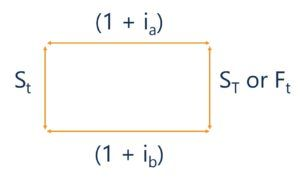### Quick Summary of Points

• The IRP demonstrates the relationship between the exchange rate and interest rates of two countries
• This relationship shows that the forward exchange rate should be equal to the spot exchange rate times the interest rate of the home country divided by the interest rate of the foreign country
• If IRP does not hold, then there is the potential to employ an arbitrage strategy

### Interest Rate Parity (IRP) Excel Calculator

Below we will go through an example question involving IRP. The calculations used to see the relationship between interest rates and the exchange rate of two countries can be effectively demonstrated through excel. To download CFI’s free  excel calculator check out the CFI Marketplace: Interest Rate Parity (IRP) Excel Calculator

### Interpreting the Interest Rate Parity (IRP)

The above diagram showcases the interest rate parity relationship. If you were to start at any corner you can see how the exchange rates and interest rates should hold so that it is not possible to profit assuming currencies are in equilibrium. Starting from the top left corner, if you were to borrow money at your home interest rate, we can see what would happen if we were to exchange this money at the spot rate, invest at the foreign interest rate, and then exchange money back into the home currency.

According to the IRP relationship, the amount that you have exchanged at the spot rate, invested in the foreign interest rate and then exchanged at the future date should be equal to simply investing in the home currency interest rate for the same period of time. The idea behind the IRP is that if currencies are in equilibrium you should not be able to profit from just exchanging money.

### Why is the Interest Rate Parity (IRP) Important?

Interest rate parity is an important concept due to the aforementioned concept. If the interest rate parity relationship does not hold, then you could make a riskless profit. The situation where IRP does not hold would allow for the use of an arbitrage strategy. For example, let us look at the scenario where the forward exchange rate is not in equilibrium with the spot exchange rate.

If the actual forward exchange rate is higher than the IRP forward exchange rate, then you could make an arbitrage profit. To do this, you would borrow money, exchange it at the spot rate, invest at the foreign interest rate and lock into the forward contract. At maturity of the forward contract, you would exchange money back into your home currency and pay back the money you borrowed to go through with this transaction. If the forward price you locked into was higher than the IRP equilibrium forward price you would be left with more than the amount you must pay back. You have essentially made riskless money out of nothing but borrowed funds.

Interest rate parity is also important in understanding exchange rate determination. Based on the IRP equation we can see how changing the interest rate can affect what we would expect the spot rate to be at a later date. For example, by holding the foreign country interest rate steady and increasing the home country’s interest rate, we would expect the home country currency to appreciate in relation to the foreign country. This would affect the expected exchange rate.

### Uncovered Interest Rate Parity vs Covered Interest Rate Parity

The uncovered and covered interest rate parities are very similar. The difference is that the uncovered IRP refers to the state in which no-arbitrage is satisfied without the use of a forward contract. In the uncovered IRP, the expected exchange rate adjusts so that IRP holds. This concept is a part of the expected spot exchange rate determination.

The covered interest rate parity refers to the state in which no-arbitrage is satisfied with the use of a forward contract. In the covered IRP, investors would be indifferent whether to invest in their home country interest rate or the foreign country interest rate since the forward exchange rate is holding the currencies in equilibrium. This concept is part of the forward exchange rate determination.

### What is the Interest Rate Parity (IRP) Equation?

The covered and uncovered IRP equation is very similar with the only difference being the substitution of the forward exchange rate for the expected spot exchange rate. The following shows the equation for the uncovered interest rate parity: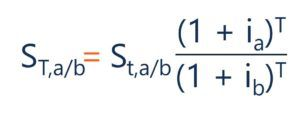ST(a/b) = St(a/b) * (1+ ia) / (1 + ib)

The following is the equation for the covered interest rate parity: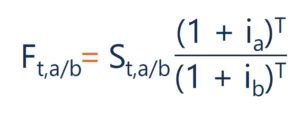Ft(a/b) = St(a/b) * (1+ ia)T / (1 + ib)T

Interest rate parity is also often shown in the form that isolates the interest rate of the home country: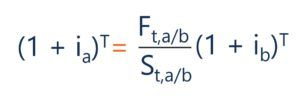For all forms of the equation:

• St(a/b) = The Spot Rate (In Currency A Per Currency B)
• ST(a/b) = Expected Spot Rate at time T (In Currency A Per Currency B)
• Ft(a/b) = The Forward Rate (In Currency A Per Currency B)
• ia = Interest Rate of Country A
• ib = Interest Rate of Country B
• T = Time to Expiration Date

### Interest Rate Parity – Worked Example

Let us now work through an example question involving the interest rate parity. Given a spot rate of 1.13\$/€, an interest rate for the \$ of 2% and an interest rate for the € of 3%, what should the one-year forward exchange rate be if the covered interest rate parity holds? The following shows the calculation to solve this question: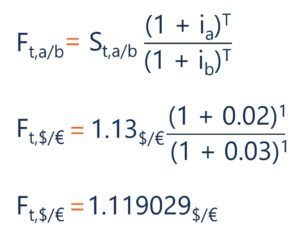The calculation to determine any component given IRP can also easily be done in excel. Below we show how CFI’s excel calculator is used to arrive at the forward exchange rate of 1.119029.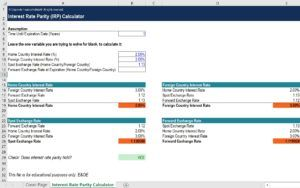Download this free calculator from CFI’s Marketplace: Interest Rate Parity (IRP) Calculator

Now that we have calculated the forward rate based on the covered interest rate parity, suppose we find out that the actual one-year forward exchange rate is 1.16\$/€. If you can borrow \$1000 payable in one year, at the home country interest rate. How much money in \$ can you make employing an arbitrage strategy?

Let us look again at the square diagram from earlier: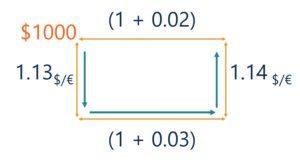Here you can see that the starting point is in the top left corner. You would borrow the \$1000, exchange it at the spot rate, lock into a forward contract and invest the money at the foreign interest rate. In one year, you could use this money to pay back the loan of \$1000*1.02 = \$1020.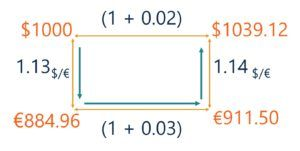Here you can see that this series of transactions would mean that after the forward contract you would be left with \$1039.12. If you were to pay back the loan of \$1020 then you would be left with: \$1039.12 – \$1020.00 = \$19.12 in profit. You have employed an arbitrage strategy and made money from nothing but borrowed funds.

Thank you for reading CFI’s article on the interest rate parity. If you would like to learn about related concepts, check out CFI’s other resources:

• Foreign Exchange
• Arbitrage
• Exchange Rate
• Put-Call Parity

### Financial Analyst Training

Get world-class financial training with CFI’s online certified financial analyst training program!

Gain the confidence you need to move up the ladder in a high powered corporate finance career path.

Learn financial modeling and valuation in Excel the easy way, with step-by-step training.